# PSEB 6th Class Maths Solutions Chapter 1 Knowing Our Numbers Ex 1.2

Punjab State Board PSEB 6th Class Maths Book Solutions Chapter 1 Knowing Our Numbers Ex 1.2 Textbook Exercise Questions and Answers.

## PSEB Solutions for Class 6 Maths Chapter 1 Knowing Our Numbers Ex 1.2

1. Convert the following measurements as directed:

Question (a)
5 km into metre
Solution:
1 km= 1000 m
∴ 5 km = 5 × 1000 m
= 5000 mQuestion (b)
35 kilometre into metre
Solution:
1 km = 1000 m
∴ 35 km = 35 × 1000 m
= 35000 m

Question (c)
2000 milligram into gram
Solution:
1000 mg = 1 gm
∴ 2000 mg = $$\frac {1}{1000}$$ × 2000 gm
= 2 gm

Question (d)
500 decigram into gram
Solution:
10 decigram = 1 gm
∴ 500 decigram = $$\frac {1}{10}$$ × 500 gm
= 50 gm

Question (e)
2000 millilitre into litre
Solution:
1000 ml = 1 litre
∴ 2000 ml = $$\frac {1}{1000}$$ × 2000 litre
= 2 litreQuestion (f)
12 kilolitre into litre
Solution:
1 kilolitre = 1000 litre
∴ 12 kilolitre = 12 × 1000 litres
= 12000 litres

2. In an election, the successful candidate registered 6317 votes whereas his nearest rival could attain only 3761 votes. By what margin did the successful candidates defeat his rival?
Solution:
Votes attained by successful candidate = 6317
Votes attained by nearest rival = 3761
Difference between their votes = 6317 – 3761 = 2556
Successful candidate defeat his rival by 2556 votes.

3. A monthly magazine having 37 pages is published on 20th day of each month. This month 23791 copies were printed. Tell us how many pages were printed in all?
Solution:
Number of pages in one copy = 37
Number of pages in 23791 copies
= 23791 × 37
= 880267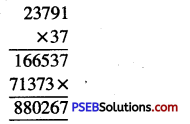4. A shopkeeper has 37 reams. One ream contain 480 pages and he wants to make quires of all these sheets to sell in retail. One quire of sheets contain 24 sheets. How many quires will be made?
Solution:
Number of pages in one ream = 480
Number of pages in 37 reams = 37 × 480
= 17760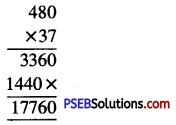Number of quires in 24 sheets = 1
Number of quires in 17760 sheets
= $$\frac {1}{24}$$ × 17760
= 740 quires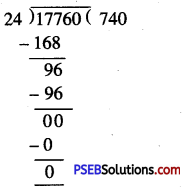5. Veerpal serves milk to the guests in glasses of capacity 250 ml each. Suppose that the glasses are filled to capacity and there was 5 litre milk that got consumed. How many guests were served with milk?
Solution:
Total quantity of milk consumed = 5 litre
= 5 × 1000 ml
= 5000 ml
Capacity of the glass = 250 ml
Number of glasses served = 5000 ÷ 250 = 20
Now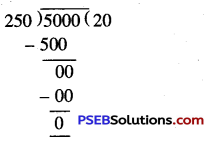The milk is served in 20 glasses6. A box of medicine contain 2,00,000 tablets each weighing 20 mg. What is the total weight of tablets inbox?
Solution:
Weight of each tablet = 20 mg
Weight of 2,00,000 tablets
= 2,00,000 × 20 mg
= 40,00,000 mg
= $$\frac {40,00,000}{1000}$$ g
= 4000 g
= $$\frac {4000}{1000}$$ = 4 kg
Hence, total weight of tablets is 4 kg

7. A bookstore sold books worth Rupees Two lakh eighty-five thousand eight hundred ninety-one in the first week of June. They sold books worth Rupees Four lakh seven hundred sixty-eight in the second week of June. How much was the total sale for two weeks together?
Solution:
Worth of books sold in first week = Rupees Two lakh eighty-five thousand eight hundred ninety one only.
= ₹ 2,85,891
Worth of books sold in second week = Rupees Four lakh seven hundred sixty-eight = ₹ 4,00,768.
Total sale for two weeks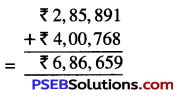8. A famous cricket player has so far scored 6978 runs in test matches. He wishes to complete 10,000 runs. How many more runs he need?
Solution:
The number of runs player wishes to complete = 10,000
The number of runs he scored = – 6,978
The number of more runs he needed = 3,022.
He needed 3,022 more runs9. Surinder has ₹ 78592 with him. He placed an order for purchasing 39 radio sets at ₹ 1234 each. How much money will remain with him after the purchase?
Solution:
Cost of one radio set = ₹ 1234
Cost of 39 radio sets = 39 × ₹ 1234
= ₹ 48126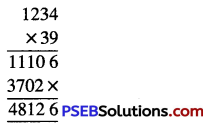Total money Surinder has = ₹ 78592
Cost of 39 radio sets = – ₹ 48126
Money remained with him = ₹ 3046610. A vessel has 3 litre 650 ml of curd. In how many glasses each of 25 ml capacity can it be distributed?
Solution:
Total quantity of curd = 3 l 650 ml
= 3 × 1000 ml + 650 ml
= 3000 ml + 650 ml
= 3650 ml
Capacity of one glass = 25 ml
Number of glasses distributed
= 3650 ÷ 25 = 146∴ The curd can be distributed in 146 glasses.